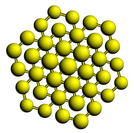Crystal Lattice Structures: Reference Date:   1 Jan 2001 Last Modified: 21 Oct 2004

# The αAs (A7) StructureYou can now

• Note that when b = 0 and u = 1/8 this structure reduces to diamond. With b = 0 and u<>1/8 this lattice can be used to determine the Raman frequency of diamond crystals via the frozen phonon approximation.
• This is the same type of structure, obviously with different structural parameters, as the rhombohedral graphite and βO2 structures, which we show in the rhombohedral orientation rather than the near cubic orientation used here.

• Prototype: αAs
• Pearson Symbol: hR2
• Strukturbericht Designation: A7
• Space Group: R3m (Cartesian and lattice coordinate listings available)
• Number: 166
• Reference: Pearson (Alloys), p. 559.
• Other Elements with this Structure: Sb, Bi, rhombohedral graphite, βO2
• Primitive Vectors:  A1 = b X + a Y + a Z A2 = a X + b Y + a Z A3 = a X + a Y + b Z
• Basis Vectors:  B1 = + u (A1 + A2 + A3) = + u (2 a + b) (X + Y + Z) (As) (2c) B2 = - u (A1 + A2 + A3) = - u (2 a + b) (X + Y + Z) (As) (2c)

### See these vectors in LaTeX output form.

Go back to the IIIb-VIIb elements structures page.

Go back to the carbon and related structures page.

Go back to Crystal Lattice Structure page.

 Structures indexed by: This is a mirror of an old page created at theNaval Research LaboratoryCenter for Computational Materials ScienceThe maintained successor is hosted at http://www.aflowlib.org/CrystalDatabase/ and published as M. Mehl et al., Comput. Mater. Sci. 136 (Supp.), S1-S828 (2017).